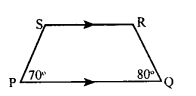# KSEEB Solutions for Class 8 Maths Chapter 15 Quadrilaterals Ex 15.2

Students can Download Maths Chapter 15 Quadrilaterals Ex 15.2 Questions and Answers, Notes Pdf, KSEEB Solutions for Class 8 Maths helps you to revise the complete Karnataka State Board Syllabus and score more marks in your examinations.

## Karnataka Board Class 8 Maths Chapter 15 Quadrilaterals Ex 15.2

Question 1.
In a trapezium PQRS, PQ || RS and ∠E= 70° and Q = 80° calculate the measure of ∠S and ∠R.∠P + ∠S = 180°
[adjacent angles correspondingto parallel sides 70° + ∠S = 180°]
∠S = 180 – 70
∠S = 110°
∠R + ∠Q = 180°
[adjacent angles corresponding to parallel sides ∠R + 80° = 180°]
∠R = 180 – 80
∠R = 100°

Question 2.
In trapezium ABCD, AB || CD. It is given that AD is not parallel to BC. Is ∆ABC ≅ ∆ADC ? Give reasons.